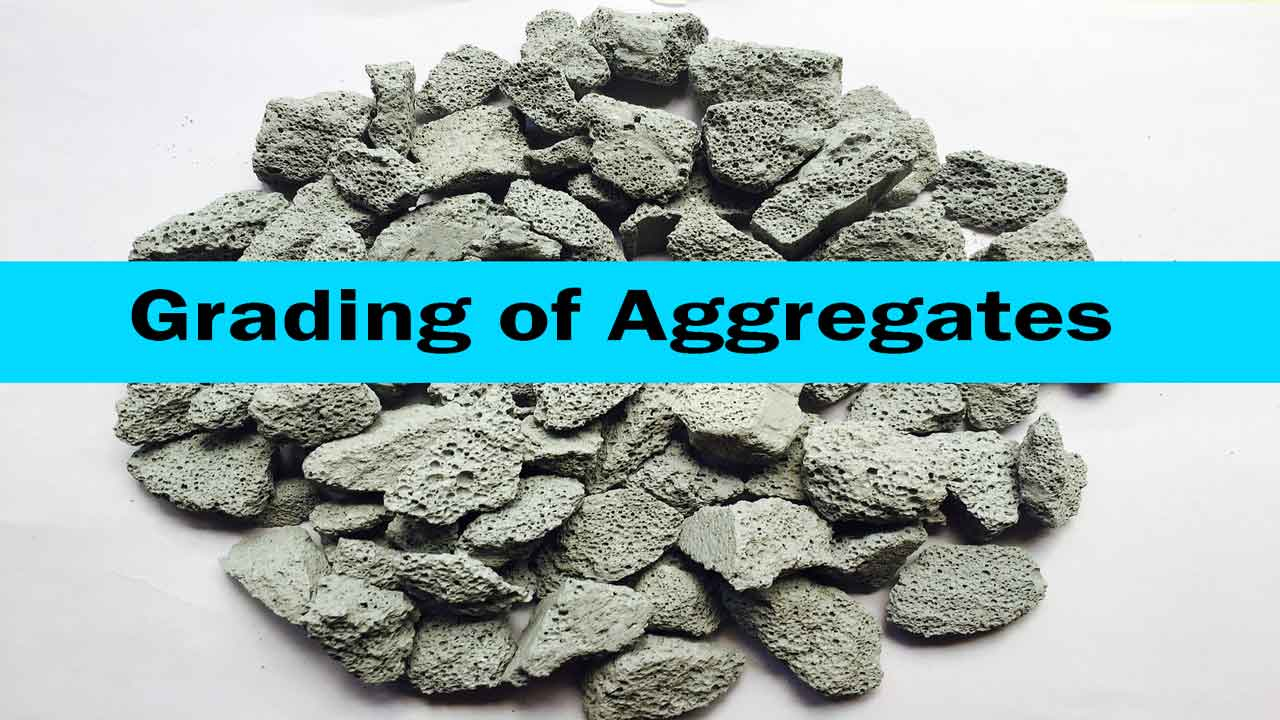# A Detailed Guide on Grading of Aggregates.

Grading of aggregates is determining the average grain size of the aggregates before they are used in construction.

This is applied to both coarse and fine aggregates. The aggregate sample is sieved through a set of sieves and weights retained on each sieve in percentage terms are summed up.

On dividing this sum by 100, The Fineness Modulus of that aggregate is determined. This helps in deciding about the quantity of aggregates of known fineness moduli to be mixed for obtaining a concrete of desired density.The basis for mixing coarse and fine aggregates of specific fineness modulus is the presence of voids or open spaces when the aggregates are packed together.

In pure coarse aggregates packing may leave 30-40 percent voids, which can be removed only by filling with finer particles.

Similarly, in fine aggregates also, voids are left that have to be filled with still finer particles (of cement).

This is essential to obtain concrete of compact and void-free character.

When some pieces of aggregates of equal size are packed together, voids or open spaces are always left. The percentage of voids may be as high as 45 percent of the total volume of aggregates.

It has been observed that this result (presence of voids) is independent of the size of aggregates used in packing, whether coarse, medium or fine.

Read More: Soundness of Cement & Its Test by Le Chatelier Apparatus.

Only, while packing aggregate should be of equal size, that is of the same grade.

Either all of them should be coarse, or all of them should be medium or fine grade. This implies that even if sand alone is packed, voids to the tune of 40-45 percent are again left.

This principle of formation of voids is the governing principle for the preparation of concrete under this method.

When the coarse aggregates are packed to make the concrete, the voids formed within the mass must be filled by some finer material. Sand is used for this purpose.

But, there will be voids left between the sand grains too. These are filled with the cement particles.

In this way, the resulting concrete mass is a void-less or dense mass. The binding property of cement is made use of to give this dense mass a cohesive stone-like character.

Concrete is, an artificial stone, in a broad sense.

Grading of aggregates is aimed at determining the mean size of the particle in a given batch of aggregates.

This is commonly found by the Method of Fineness Modulus. The method can be used to determine fineness modulus of coarse aggregates, fine aggregates, and all-in aggregates, i.e., mixed aggregates.

In this method, a convenient weight of the sample is taken and sieved through a set of sieves one after another. The number of sieves is five for the coarse aggregates and ten for all-in-aggregates.

It is only six in the case of fine aggregates.

Sieve Size for Grading of Aggregates.

Coarse Aggregates: 80 mm, 40 mm, 20 mm, 10 mm, IS Nos. 480

Fine Aggregates: IS No. 480, 240, 120, 60, 30 and 15.

All in aggregates: 80 mm, 40 mm, 20 mm, 10 mm, Nos: 480, 240, 120, 60, 30 and 15.

Read More: Top 12 Different Types of Cranes used in Construction Works.

It will be noted that each successive sieve has the diameter of mesh reduced to 50 percent.

Calculations involve dividing the cumulative percentage of weights retained on these set of sieves by 100.

The resulting figure gives fineness modulus of the respective aggregate. This is illustrated in the following example.

A weight of 10 kg of Coarse aggregate and 5 kg of fine aggregate has been taken to determine fineness modulus in each case separately.

Let us assume that the weight of aggregate retained in each case and the calculated cumulative percentage of retained weights are as follows:

IS Sieve No.Weight RetainedTotal Weight (kg)Retained Weight (gm)
80 mm000
40 mm000
20 mm2220
10 mm4660
4803.59.595
2400.510100
120100
60100
30100
15100
Total10 Kg6.75 Kg

Cumulative Percentage of weight retained on all the ten sieves – 675 Fineness modulus of coarse aggregate = 675/100 = 6.75 kg.

IS Sieve No.Weight retainedTotal Weight (kg)Retained (gm)
4800.20.24
2400.81.020
1200.51.530
601.22.754
301.54.284
150.85.0100
Total5 kg2.92 kg

Cumulative percentage of weight retained on all the six sieves = 292 Fineness Modulus of Fine Aggregates = 292/100 =2.92 kg.

Now, when it is desired to obtain a combined aggregate of a definite (required) fineness modulus, F, the amount of fine aggregate (x) to be mixed with 1 volume of the coarse aggregate of a given fineness modulus can be easily determined from the relationship:

x=Fc – F / F – Ff

Where “x” is the amount of fine aggregate (by volume)for 1 volume of coarse aggregate.

Fc = Fineness modulus of coarse aggregate.

F = Fineness modulus of desired mixed aggregate.

Ff = Fineness modulus of fine aggregate.

The above relationship is illustrated in the following example.

F = 5.5

Fc = 6.75

Ff = 2.92

To Determine: Volume of fine aggregate (x) in percentage terms of volume of coarse aggregate.

x=Fc – F / F – Ff

=> 6.75 – 5.3 / 5.5-2.92 x 100

=> 48.5 % approximately.

### Limits of Fineness.

Repeated trials with mix designs using different aggregates have shown that following limits of fineness moduli hold good for obtaining concrete mixes of good workability.

Cement Consumption is also reasonable when limits are followed.

Aggregate TypeMaximum Size (mm)Recommended Moduli Maximum.Recommended Moduli Manimum.
Fine2.003.5
Coarse2066.90
406.907.50
807.508.00
1608.008.50
Mixed204.705.10
255.105.5
325.25.7
405.45.9
805.86.3
160657.0

The upper limit of fineness modulus is, therefore, always below 8.5 for coarse and 7.0 for mixed aggregates.

1.